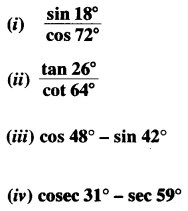NCERT Solutions for Class 10 Maths Chapter 8 Introduction to Trigonometry Ex 8.3 are part of NCERT Solutions for Class 10 Maths. Here we have given NCERT Solutions for Class 10 Maths Chapter 8 Introduction to Trigonometry Ex 8.3.

 Board CBSE Textbook NCERT Class Class 10 Subject Maths Chapter Chapter 8 Chapter Name Introduction to Trigonometry Exercise Ex 8.3 Number of Questions Solved 7 Category NCERT Solutions

## NCERT Solutions for Class 10 Maths Chapter 8 Introduction to Trigonometry Ex 8.3

Question 1.Solution:Question 2.
Show that:
(i) tan 48° tan 23° tan 42° tan 67° = 1
(ii) cos 38° cos 52° – sin 38° sin 52° = 0
Solution:
(i) LHS = tan 48° tan 23° tan 42° tan 67°
= tan 48° tan 23° tan (90° – 48°) tan (90° – 23°)
= tan 48° tan 23° cot 48° cot 23° = tan 48° tan 23° .$$\frac{1}{\tan 48^{\circ}} \cdot \frac{1}{\tan 23^{\circ}}$$
= 1 = RHS

(ii) LHS = cos 38° cos 52° – sin 38° sin 52°
= cos 38° cos (90° – 38°) – sin 38° sin (90° – 38°)
= cos 38° sin 38°- sin 38° cos 38° = 0 = RHS

Question 3.
If tan 2A = cot (A – 18°), where 2A is an acute angle, find the value of A.
Solution:
tan 2A = cot (A – 18°)
⇒ cot (90° – 2A) = cot (A – 18°) [∵cot (90° – θ) = tan θ]
⇒ 90° – 2A = A – 18° ⇒ 3A = 108° ⇒ A = $$\frac { 108° }{ 3 }$$
∴ ∠ A = 36°

Question 4.
If tan A = cot B, prove that A + B = 90°.
Solution:
tan A = cot B ⇒ tan A = tan (90° – B) [ ∵ tan (90° – θ) = cot θ]
⇒ A = 90° – B ⇒ A + B = 90° Proved

Question 5.
If sec 4A = cosec (A – 20°), where 4A is an acute angle, find the value of A.
Solution:
sec 4A = cosec (A – 20°)
⇒ cosec (90° – 4A) = cosec (A – 20°) [cosec (90° – θ) = sec θ]
⇒ 90° – 4A = A – 20° ⇒ 5A = 110°
A = $$\frac { 110° }{ 5 }$$
A = 22°
∴ ∠ A = 22°

Question 6.
If A, Band Care interior angles of a triangle ABC, then show that: sin ($$\frac { B+C }{ 2 }$$) = cos $$\frac { A }{ 2 }$$
Solution:Question 7.
Express sin 61° + cos 75° in terms of trigonometric ratios of angles between 0° and 45°.
Solution:
sin 67° + cos 75° = sin (90° – 23°) + cos (90° – 15°) = cos 23° + sin 15°

We hope the NCERT Solutions for Class 10 Maths Chapter 8 Introduction to Trigonometry Ex 8.3, help you. If you have any query regarding NCERT Solutions for Class 10 Maths Chapter 8 Introduction to Trigonometry Ex 8.3, drop a comment below and we will get back to you at the earliest.